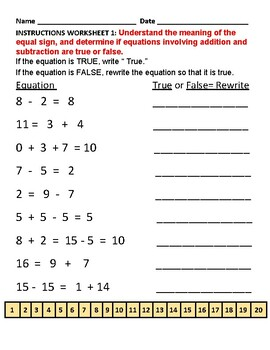Grade 1- Operations and Algebraic Thinking for Traditional Students - CCSSubject
Resource Type
Common Core State Standards
Product Rating
4.0
1 Rating
File Type

PDF (Acrobat) Document File

Be sure that you have an application to open this file type before downloading and/or purchasing.

614 KB|12 pages
Share
Also included in:
1. For First Grade Traditional Students Instructors who use this variety of instructional modes will enhance learning for traditional students. This educational resource pack includes:  tactile and visual prompts applied.   Accessibility suggestions: Go to your device “Settings” where you can adjust
\$17.65
\$12.35
Save \$5.30
2. \$8.85
\$7.08
Save \$1.77
3. For Grade 1 Traditional Students Accessibility suggestions: Go to your device “Settings” where you can adjust the screen’s: brightness and color, size of text and resolution, and connect to wireless display and adjust sound.This educational resource pack includes the following classroom subjects:
\$80.45
\$74.16
Save \$6.29
Product Description

“Operations and Algebraic Thinking” - First Grade Math

This educational resource pack includes: Understanding addition as putting together and adding to, and understand subtraction as taking apart and taking from. Represent addition and subtraction with objects, fingers, mental images, sounds (e.g., claps), acting out situations, verbal explanations, expressions, or equations.

Operations and Algebraic Thinking - represent and solve problems involving addition and subtraction.

Use addition and subtraction within 20 to solve word problems involving situations of adding to, taking from, putting together, taking apart, and comparing, with unknowns in all positions, e.g., by using objects, drawings, and equations with a symbol for the unknown number to represent the problem.

Solve word problems that call for addition of three whole numbers whose sum is less than or equal to 20, e.g., by using objects, drawings, and equations with a symbol for the unknown number to represent the problem. understand and apply properties of operations and the relationship between addition and subtraction.

Apply properties of operations as strategies to add and subtract. (Note: Students need not use formal terms for these properties.) Examples: If 8 + 3 = 11 is known, then 3 + 8 = 11 is also known. (Commutative property of addition.) To add 2 + 6 + 4, the second two numbers can be added to make a ten, so 2 + 6 + 4 = 2 + 10 = 12. (Associative property of addition.)

Understand subtraction as an unknown-addend problem. For example, subtract 10 – 8 by finding the number that makes 10 when added to 8. add and subtract within 20.

Relate counting to addition and subtraction (e.g., by counting on 2 to add 2).

Add and subtract within 20, demonstrating fluency for addition and subtraction within 10. Use strategies such as counting on; making ten (e.g., 8 + 6 = 8 + 2 + 4 = 10 + 4 = 14); decomposing a number leading to a ten (e.g., 13 – 4 = 13 – 3 – 1 = 10 – 1 = 9); using the relationship between addition and subtraction (e.g., knowing that 8 + 4 = 12, one knows 12 – 8 = 4); and creating equivalent but easier or known sums (e.g., adding 6 + 7 by creating the known equivalent 6 + 6 + 1 = 12 + 1 = 13). Work with addition and subtraction equations.

Understand the meaning of the equal sign, and determine if equations involving addition and subtraction are true or false. For example, which of the following equations are true and which are false? 6 = 6, 7 = 8 – 1, 5 + 2 = 2 + 5, 4 + 1 = 5 + 2.

Here is a link for our teaching strategies bundle for students of all ability levels:

Here is a link to additional worksheets for traditional students : https://www.teacherspayteachers.com/My-Products/Category:356865/sort:Item.rating/direction:desc

Click here to Stone Soup School and our new products:

Help us by taking a moment to rate this product.

Thank You!

Total Pages
12 pages
N/A
Teaching Duration
90 minutes
Report this Resource to TpT
Reported resources will be reviewed by our team. Report this resource to let us know if this resource violates TpT’s content guidelines.